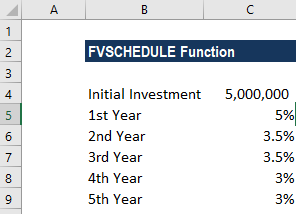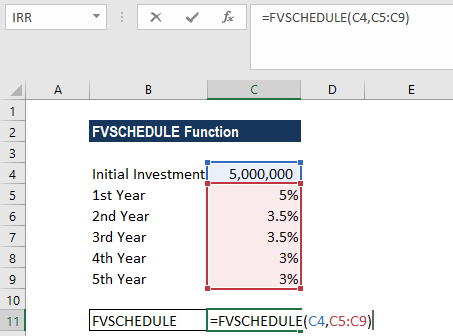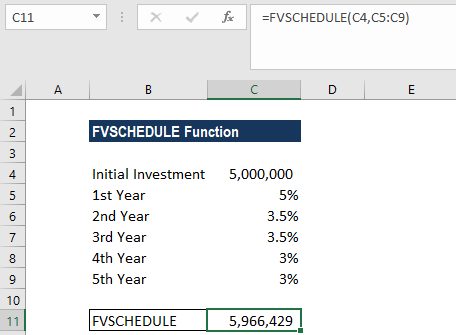# FVSCHEDULE Function

Calculates the future value of an investment with a variable interest rate

## What is the FVSCHEDULE Function?

The FVSCHEDULE function is categorized under Excel Financial functions. It will calculate the future value of an investment with a variable interest rate.

In financial analysis, we often need to make a decision on investments made by a company. Sometimes, we make investments that will guarantee a certain percentage for the first year, say 5%, 6% on the second year, etc. In such a scenario, we need to evaluate the investment, which can be done using FVSCHEDULE function.

### Formula

=FVSCHEDULE(principal, schedule)

The FVSCHEDULE function uses the following arguments:

1. Principal (required argument) – This is the present value of the investment.
2. Schedule (required argument) – This is an array of values that provides the schedule of interest rates to be applied to the principal.

If provided as a range of cells, they may contain numeric values or may be empty (empty cells denote a 0% interest rate).

### How to use the FVSCHEDULE Function in Excel?

To understand the uses of the FVSCHEDULE function, let’s consider an example:

#### Example

Suppose we invest \$5 million that will give 5% in the first year, 3.5% in the next two years and 3% in the remaining years. Let’s calculate the future value using the FVSCHEDULE function:The formula to use is:In the formula, we will first provide the initial investment and a schedule of interest rates.

The result will be:The future value of our investment is \$5,966,429.

### Few notes about the FVSCHEDULE Function

1. #VALUE! error – Occurs when any of the given arguments is non-numeric.
2. The FVSCHEDULE function accepts blank cells as a part of the scheduled array as they are treated as the numeric value 0. They are not interest payable.
3. Remember that if we enter the interest rates directly into the function instead of cell references, we need to enter the interest rates as an array of values. For example, FVSCHEDULE (B3, {0.025, 0.030, 0.040, 0.045}).
4. If the interest rates are constant and if we are given the nper, pmt and/or pv and type arguments, we must use the FV function to calculate the future value of an investment.
5. FVSCHEDULE was introduced in MS Excel 2007 and hence not available in earlier versions.

Thanks for reading CFI’s guide to important Excel functions! By taking the time to learn and master these functions, you’ll significantly speed up your financial analysis. To learn more, check out these additional CFI resources:

• Excel Functions for Finance